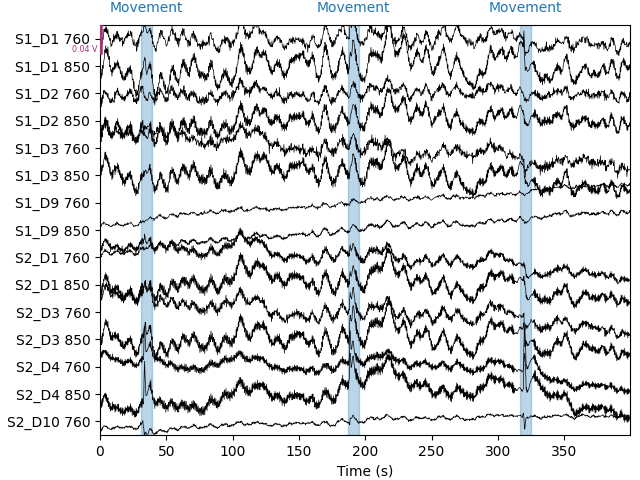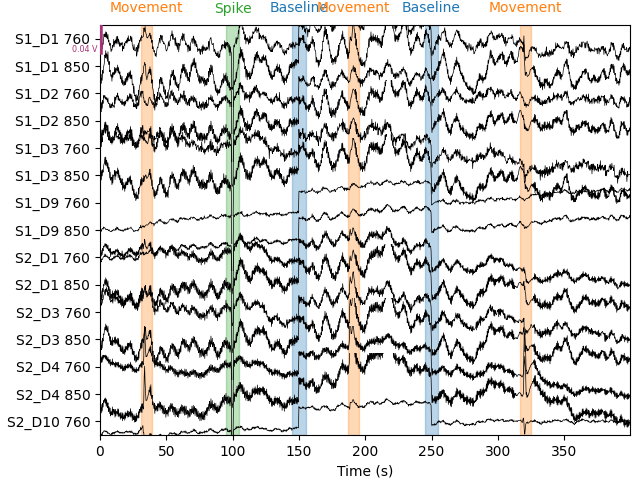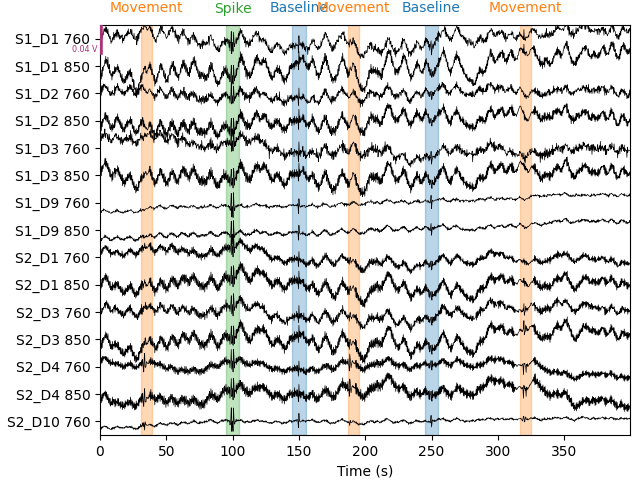Visualise NIRS artifact correction methods¶

Here we artificially introduce several fNIRS artifacts and observe how artifact correction techniques attempt to correct the data.

# Authors: Robert Luke <mail@robertluke.net>
#

import os
import mne

from mne.preprocessing.nirs import (optical_density,
temporal_derivative_distribution_repair)

Import data¶

Here we will work with the fNIRS motor data. We resample the data to make indexing exact times more convenient. We then convert the data to optical density to perform corrections on and plot these signals.

fnirs_data_folder = mne.datasets.fnirs_motor.data_path()
fnirs_cw_amplitude_dir = os.path.join(fnirs_data_folder, 'Participant-1')
raw_od = optical_density(raw_intensity)
new_annotations = mne.Annotations([31, 187, 317], [8, 8, 8],
["Movement", "Movement", "Movement"])
raw_od.set_annotations(new_annotations)
raw_od.plot(n_channels=15, duration=400, show_scrollbars=False)Out:

Reading 0 ... 23238  =      0.000 ...  2974.464 secs...

We can see some small artifacts in the above data from movement around 40, 190 and 240 seconds. However, this data is relatively clean so we will add some additional artifacts below.

Two common types of artifacts in NIRS data are spikes and baseline shifts. Spikes often occur when a person moves and the optode moves relative to the scalp and then returns to its original position. Baseline shifts occur if the optode moves relative to the scalp and does not return to its original position. We add a spike type artifact at 100 seconds and a baseline shift at 200 seconds to the data.

corrupted_data = raw_od.get_data()
corrupted_data[:, 298:302] = corrupted_data[:, 298:302] - 0.06
corrupted_data[:, 450:750] = corrupted_data[:, 450:750] + 0.03
corrupted_od = mne.io.RawArray(corrupted_data, raw_od.info,
first_samp=raw_od.first_samp)
new_annotations.append([95, 145, 245], [10, 10, 10],
["Spike", "Baseline", "Baseline"])
corrupted_od.set_annotations(new_annotations)

corrupted_od.plot(n_channels=15, duration=400, show_scrollbars=False)Out:

Creating RawArray with float64 data, n_channels=56, n_times=8924
Range : 0 ... 8923 =      0.000 ...  2974.333 secs